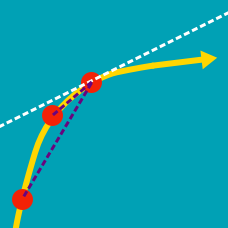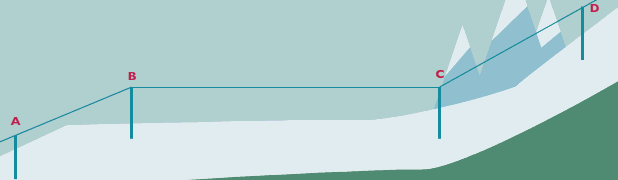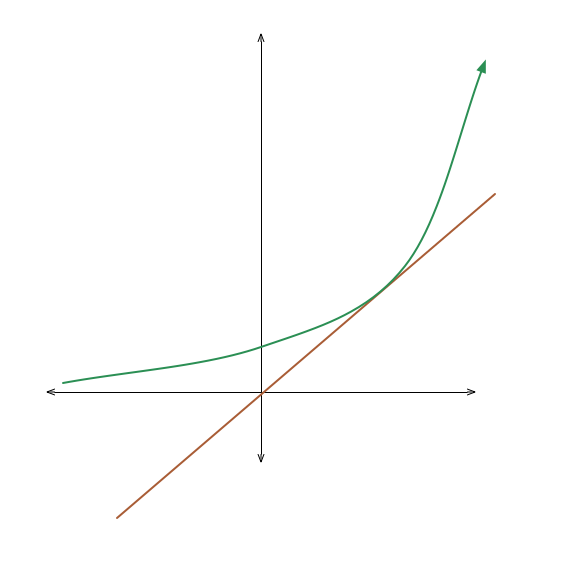Calculus

Derivatives: Level 2 ChallengesSki lifts are an important cable transport that helps to carry skiers up a hill, so that they can easily enjoy skiing down the hill.

Which portion of the ski lift is the steepest?

We know that $\dfrac d{dx} x^n = n x^{n-1}$.

Is it also true that $\dfrac d{dx} n^x = x n^{x-1}$?

$\large y_n (x) = e^x \times e^{x^2} \times e^{x^3} \times \ldots \times e^{x^n}$

For some positive integer $n$, let $y_n(x)$ denote function of $x$ as stated above.

What is the value of the limit below?

$\large \lim_{n\to\infty} \left . \frac d{dx} \left ( y_n (x) \right ) \right |_{x=\frac12}$Let $a \gt 1$ be the unique real number such that the equation $a^{x} = x$ has a unique solution. Compute $\lceil 1000a \rceil$.

$\large\displaystyle\lim_{(x,y,z) \to (0,0,0)} \dfrac{e^{-x^{2} - y^{2} - z^{2}} - 1}{x^{2} + y^{2} + z^{2}} =\, ?$

×